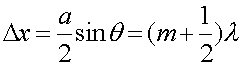Single-slit diffractionA single slit, as shown on the right, also causes a diffraction pattern. This results because the slit is of finite width, and every point in the slit serves as a source of a wave. Consider a slit of width a where we will consider two points of emission, 1 and 2, one from the upper half of the slit and one from the lower half. The points will be separated by a/2. For light that travels in the direction q, the contribution from point 1 will cancel the contribution from the point 2 if the difference Dx is a half-integral number of wavelengths. Thus, there is a MINIMUM for angles,For the first minimum, m = 1, the formula looks exactly like the expression for the maximum for the two-slit problem, only that one is refering to the slit width a here, whereas we refered to the slit separation d in the two-slit problem.

Examples     Interference and diffraction's index